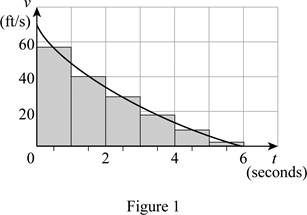# The distance traveled by the car when the brakes are applied.### Single Variable Calculus: Concepts...

4th Edition
James Stewart
Publisher: Cengage Learning
ISBN: 9781337687805### Single Variable Calculus: Concepts...

4th Edition
James Stewart
Publisher: Cengage Learning
ISBN: 9781337687805

#### Solutions

Chapter 5.1, Problem 15E
To determine

## The distance traveled by the car when the brakes are applied.

Expert Solution

The distance traveled by the car is 155 ft.

### Explanation of Solution

Given information:

The velocity with respect to time is a decreasing curve.

The curve lies in the interval between t=0 and t=6sec.

Use right endpoints as a lower estimate, left end points as an upper estimate, and mid endpoints as mid estimate due to a decreasing velocity curve with respect to time.

The expression to find the distance using mid estimate (Mn) is shown below:

Mn=v(t1)Δt+v(t2)Δt+...+v(tn)Δt (1)

Here, the mid height of the first rectangle is v(t1), the time interval is Δt,  the mid height of second rectangle is v(t2), and the mid height of nth rectangle is v(tn).

Take interval Δt as 1 sec and the number of rectangles as 6.

Draw six rectangles using mid endpoints for Δt=1 as shown below in Figure (1).Refer Figure (1).

Take the mid height of the first rectangle’s v(t1) value as 55ft/sec, the mid height of second rectangle’s v(t2) value as 40ft/sec, the mid height of third rectangle’s v(t3) value as 28ft/sec, the mid height of fourth rectangle’s v(t4) value as 18ft/sec, the mid height of fifth rectangle’s v(t5) value as 10ft/sec, and the mid height of sixth rectangle’s v(t6) value as 4ft/sec.

Substitute 6 for n, 55ft/sec for v(t1), 1sec for Δt, 40ft/sec for v(t2), 28ft/sec for v(t3), 18ft/sec for v(t4), 10ft/sec for v(t5) and 4ft/sec for v(t6) in Equation (1).

M6=(55×1)+(40×1)+(28×1)+(18×1)+(10×1)+(4×1)=55+40+28+18+10+4=155ft.

Therefore, the mid estimate using mid endpoints for n=6 is 155 ft.

Check whether the estimated value is reasonable or not:

Find the area of triangle (A) as shown below:

A=12×b×h (2)

Here, the width of triangle is b and the height of the triangle is h.

Refer to Figure (1).

Assume the curve as a triangle by drawing a line from (6,0) to (0,70).

Take the width of triangle b value as 6 sec and the height of the triangle h value as 70 ft/sec.

Substitute 6 sec for b and 70 ft/sec for h in Equation (2).

A=12×6×70=210ft

The assumed triangle value is an overestimate compared to the calculated mid estimate value as 155 ft.

210ft>150ft.

So the calculated mid estimate is a reasonable value.

Hence, the distance travelled by the car is 155 ft when the brakes are applied.

### Have a homework question?

Subscribe to bartleby learn! Ask subject matter experts 30 homework questions each month. Plus, you’ll have access to millions of step-by-step textbook answers!